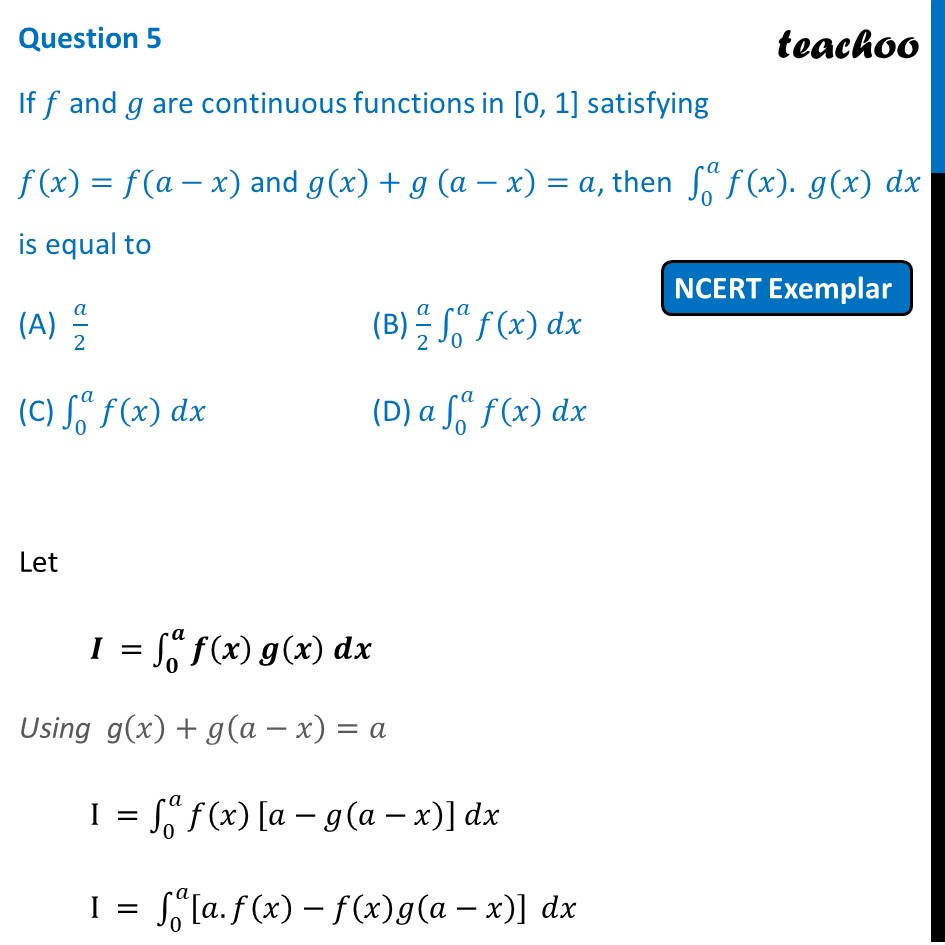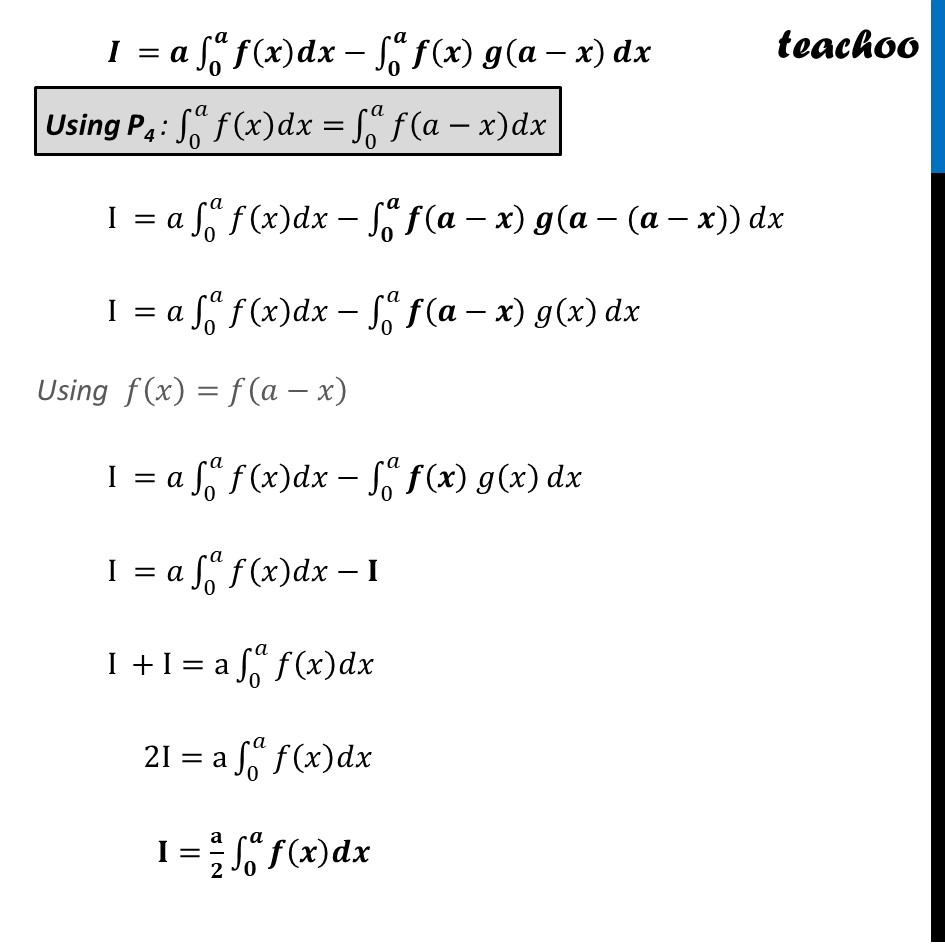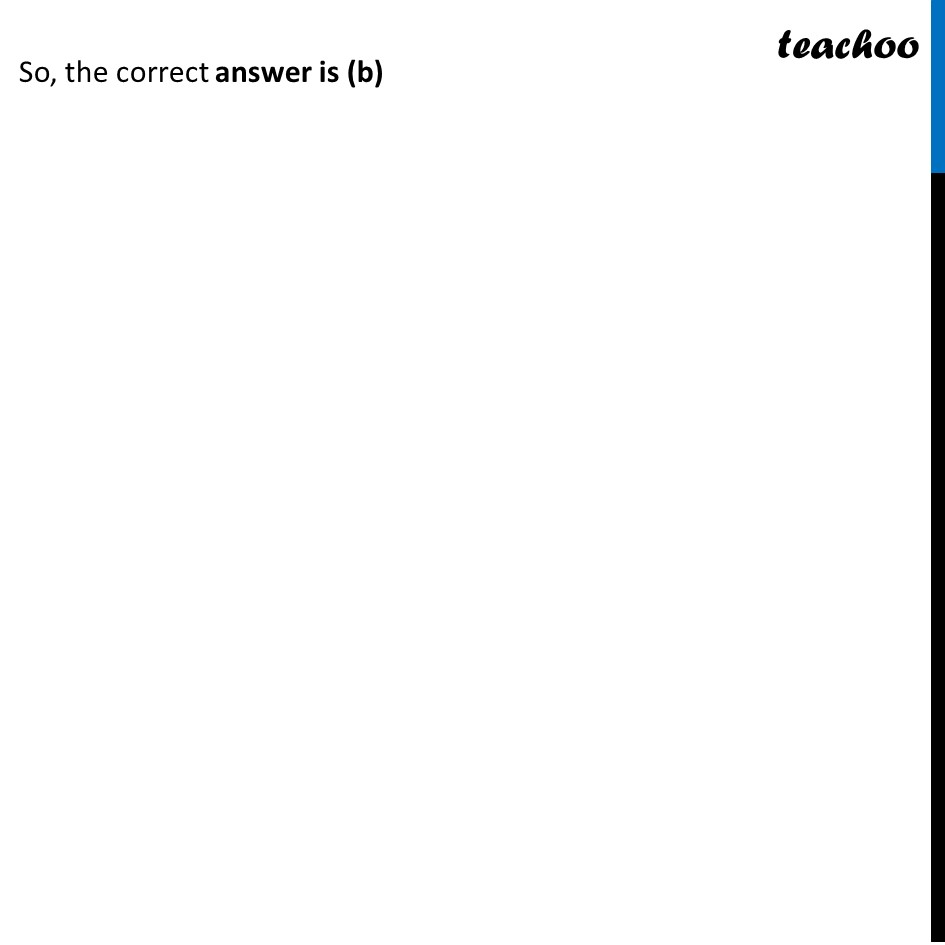NCERT Exemplar MCQ

Chapter 7 Class 12 Integrals
Serial order wise

## (D) a∫ 0^a f(x) dx

This question is similar to Ex 7.11, 19 - Chapter 7 Class 12 - IntegralsLearn in your speed, with individual attention - Teachoo Maths 1-on-1 Class

### Transcript

Question 5 If 𝑓 "and" 𝑔 are continuous functions in [0, 1] satisfying 𝑓(𝑥)=𝑓(𝑎−𝑥) and 𝑔(𝑥)+𝑔 (𝑎−𝑥)=𝑎, then ∫1_0^𝑎▒〖𝑓(𝑥). 𝑔(𝑥)〗 𝑑𝑥 is equal to 𝑎/2 (B) 𝑎/2 ∫1_0^𝑎▒〖𝑓(𝑥) 𝑑𝑥〗 (C) ∫1_0^𝑎▒〖𝑓(𝑥) 𝑑𝑥〗 (D) 𝑎∫1_0^𝑎▒〖𝑓(𝑥) 𝑑𝑥〗 Let 𝑰 =∫_𝟎^𝒂▒𝒇(𝒙) 𝒈(𝒙) 𝒅𝒙 Using g(𝑥)+𝑔(𝑎−𝑥)=𝑎 I =∫_0^𝑎▒𝑓(𝑥) [𝑎−𝑔(𝑎−𝑥)] 𝑑𝑥 I = ∫_0^𝑎▒[𝑎.𝑓(𝑥)−𝑓(𝑥)𝑔(𝑎−𝑥)] 𝑑𝑥 𝑰 =𝒂∫_𝟎^𝒂▒〖𝒇(𝒙)𝒅𝒙−∫_𝟎^𝒂▒〖𝒇(𝒙) 𝒈(𝒂−𝒙) 〗〗 𝒅𝒙 I =𝑎∫_0^𝑎▒〖𝑓(𝑥)𝑑𝑥−∫_𝟎^𝒂▒〖𝒇(𝒂−𝒙) 𝒈(𝒂−(𝒂−𝒙)) 〗〗 𝑑𝑥 I =𝑎∫_0^𝑎▒〖𝑓(𝑥)𝑑𝑥−∫_0^𝑎▒〖𝒇(𝒂−𝒙) 𝑔(𝑥) 〗〗 𝑑𝑥 Using 𝑓(𝑥)=𝑓(𝑎−𝑥) I =𝑎∫_0^𝑎▒〖𝑓(𝑥)𝑑𝑥−∫_0^𝑎▒〖𝒇(𝒙) 𝑔(𝑥) 〗〗 𝑑𝑥 I =𝑎∫_0^𝑎▒〖𝑓(𝑥)𝑑𝑥−𝐈〗 I +I=a∫_0^𝑎▒𝑓(𝑥)𝑑𝑥 2I=a∫_0^𝑎▒𝑓(𝑥)𝑑𝑥 𝐈=𝐚/𝟐 ∫_𝟎^𝒂▒𝒇(𝒙)𝒅𝒙 ∴ ∫_0^𝑎▒〖𝑓(𝑥) 𝑔(𝑥) 〗 𝑑𝑥=2∫_0^𝑎▒𝑓(𝑥)𝑑𝑥 Hence Proved So, the correct answer is (b)